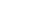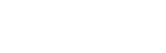## Mathematics Questions and Answers - Form 1 Mid Term 2 Exams 2021

MATHEMATICS
FORM 1
MID TERM 2

INSTRUCTIONS:• This paper has 2 sections: I and II

SECTION I
Answer all the questions from this section

1. Write the following numbers in figures
1. Ten thousands and ten (1 mark)One hundred and two billion, three hundred and sixty five million, four hundred and seventy eight thousands and one (1 mark)
2. All prime numbers less than ten are arranged in descending order to form a number.
1. Write down the number formed. (1 mark)
2. State the total value of the second digit in the number formed in (a) above (1 mark)
3. Express 7056 as a product of its prime factors leaving your answer in power form. (3 marks)
4. Three bells ring at an interval of 9 minutes, 15 minutes and 21 minutes. The bells will next ring together at 11.00pm. Find the time the bells had last rang together? (3marks)
5. Three tanks are capable of holding 108 litres, 168 litres and 180 litres of milk. Determine the capacity of the greatest vessel which can be used to fill each one of them an exact number of times. (3 marks)
6. Test whether 1 478 019 is divisible by 11. (2 marks)
7. Evaluate. (3 marks)
-16 ÷4+6 ×14-2 × -5
84÷14 ×3
8. Evaluate (2 marks)
13 of (5/- 1/4) ÷ 1/12
9. Express the recurring decimal below as a fraction in simplest form. (3 marks)10. Evaluate using tables of square roots (3 marks)
√0.792
11. Without using mathematical tables or calculator evaluate (4 marks)
0.0625×2.56
0.25×0.08×0.5

SECTION II (20 MARKS)
Answer ALL the questions from this section1. Kinyua spent 1/4 of his net January salary on school fees. He spent 1/4 of the remainder on electricity and water bills. He spent 1/9 of what remained on transport. If he finally has sh.8400, calculate
1. His total January salary. (4 marks)
2. Money spent on school fees. (2 marks)
3. Money spent on transport. (2 marks)
4. Money spent on electricity and water bills. (2 marks)
2. A bookstore has 30 816 exercise books which were packed in cartons. Each carton contained 24 exercise books. The mass of an empty carton was 2kg and that of a full carton is 12kg.
1. How many cartons ware there? (2 marks)
2. What was the total mass of the empty cartons? (2 marks)
3. What was the total mass of books in one carton? (2 marks)
4. What was the total mass of all the exercise books? (2 marks)
5. What was the mass of one exercise book in kg to 2 decimal places? (2 marks)

MARKING SCHEME

1.
1. 10, 010
2. 102 365 478 001
2.
1. 7 532
2. Total value = 5 x 100 = 500
3. 7056
 2 7056 2 3528 2 1764 2 882 3 441 3 147 7 49 7 7 1
7056 = 2 x 2 x 2 x 2 x 3 x 3 x7 x 7
= 24 x 32 x 72

4.  3 9 15 21 3 3 5 7 5 1 5 7 7 1 1 1 1 1 1
7x5x3x3 = 315 minutes = 315/60=5 hrs 15 minutes
11.00 pm – 5 hrs 15 minutes
10.45 p.m

5.
 2 108 168 180 2 54 84 90 3 27 42 45 9 14 15
Largest container = 2 x 2 x 3
= 12 litres

6. 1 478 019
(1+7+0+9) – (4+8+1)
17 – 13 =4
Therefore 1 478 019 is not divisible by 11

7. NUMERATOR
-4 + 84 + 10
=90
DENOMINATOR
6 X 3 = 18
QUOTIENT
90/18=5

8. 1/3 of 7/12 ÷ 1/12
1/3 X 7/12= 7/36
7/36 ÷ 1/12 = 7/36 × 12/1= 7/3
2 1/3

9. Let x=0.2333333333
10x = 2.333333...
100x = 23.333333...
100x – 10x = 23.333333...– 2.333333...
90x = 21
x= 21/90 = 7/30

10. √0.792= √79.2×10-2
= 8.899 × 1/10
=0.8899

11.= √625×256
25×8×50
=√16
=4

12.
1. School fees = 1/4
Remainder = 4/4 - 1/4= 3/4
Electricity and water = 1/4 of 3/4= 1/4 × 3/4= 3/16
Fees + electricity and water = 1/+ 3/16= 7/16
Remainder = 16/1- 7/16= 9/16
Transport = 1/9 of 9/16 = 1/9 × 9/16= 1/16
Fees + electricity and water+transport
= 1/4 + 3/16 + 1/16= 8/16=1/2
Remaining = 1/2=8400
Total January salary = 2/1 ×8400=shs.16 800

2. School fees
=1/4 ×16800
=shs. 4200

3. Transport
=1/16 ×16800
=shs. 1050

4. Water and electricity
=3/16 ×16800
=shs. 3150
13.
1. Number of cartons
=30 816/24
=1284 cartons

2. Total mass of the empty cartons
1284 cartons×2kg
2568kg

3. Total mass of books in one carton
mass of books in one carton=12kg-2 kg
=10kg per carton

4. Total mass of all the exercise books
Total mass of all the exercise books=1284 10kg
=12 840 kg

5. Mass of one exercise book
mass of one book =10kg ÷24
= 0.42kg